# Create animations of your design process

The main purpose of `{camcorder}` is the recording of all steps involved in a data visualization design with the ultimate goal to generate an animated gif file of all steps. (For more on the pretty cool side effects for your workflow thanks to `{camcorder}` look here).

## Start a Recording with `gg_record()`

We initialize a recording with the `gg_record()` function. After running the following code, `{camcorder}` is saving a file with the given specifications in the given directory every time `ggplot()` is called.

``````library(camcorder)

gg_record(
dir = file.path(tempdir(), "recording"),
device = "png", # we need to set the Cairo device
width = 8,
height = 5
)``````

Note: If you want to keep your plot files afterwards, set the `dir` in `gg_record` to a permanent directory (instead of a temporary directory as in our examples).

Now we can start building our plot:

``````ggplot(diamonds, aes(x = carat, y = price)) +
geom_point()``````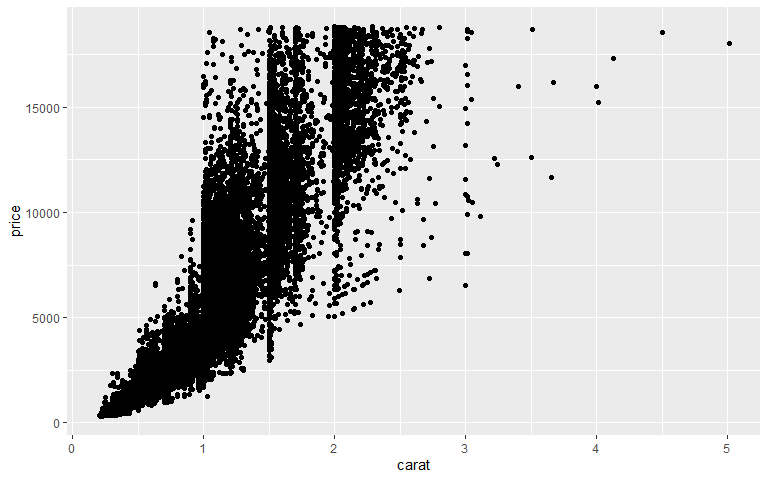``````
ggplot(diamonds, aes(x = carat, y = price)) +
geom_point(alpha = .2)``````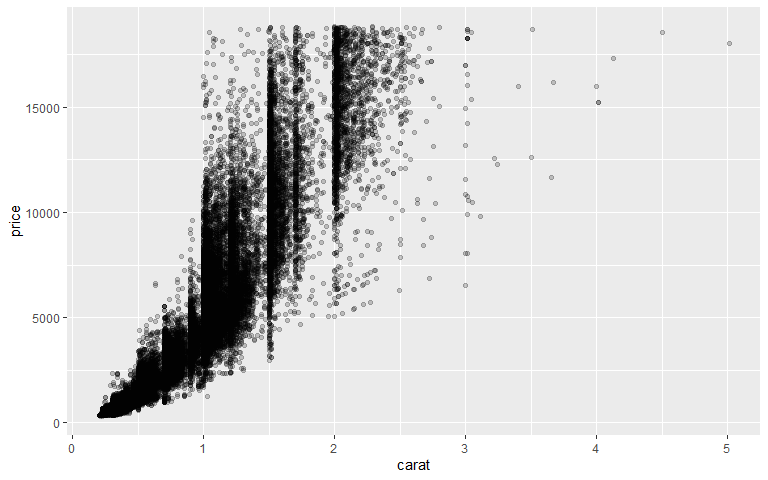``````
ggplot(diamonds, aes(x = carat, y = price)) +
geom_bin2d()``````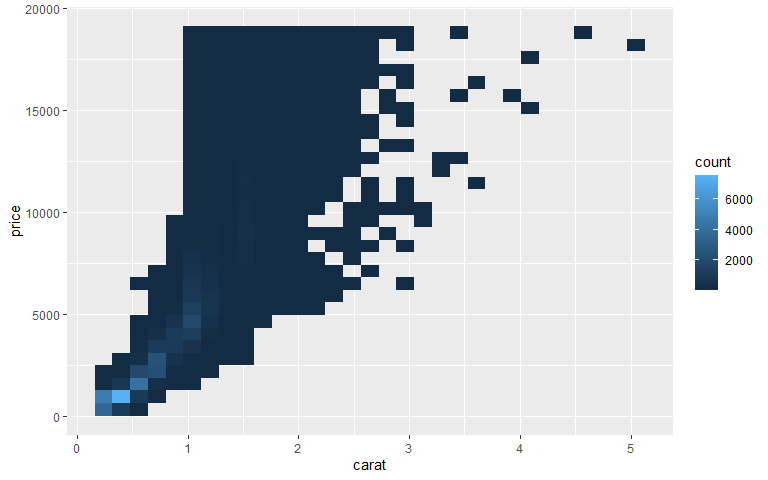``````
ggplot(diamonds, aes(x = carat, y = price)) +
geom_hex()``````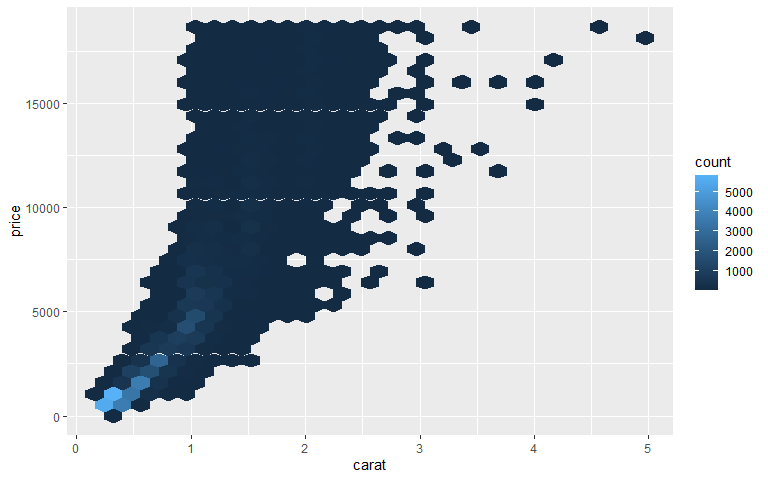``````
ggplot(diamonds, aes(x = carat, y = price)) +
geom_hex() +
scale_fill_viridis_c(option = "magma")``````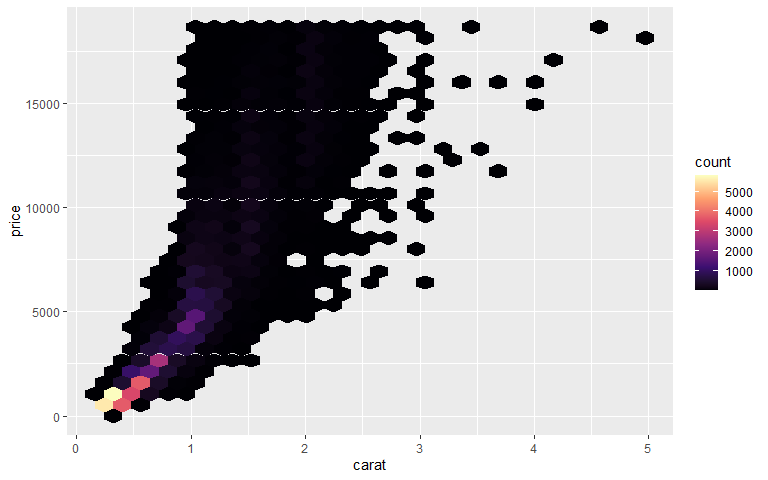``````
ggplot(diamonds, aes(x = carat, y = price)) +
geom_hex() +
scale_fill_viridis_c(option = "magma", direction = -1)``````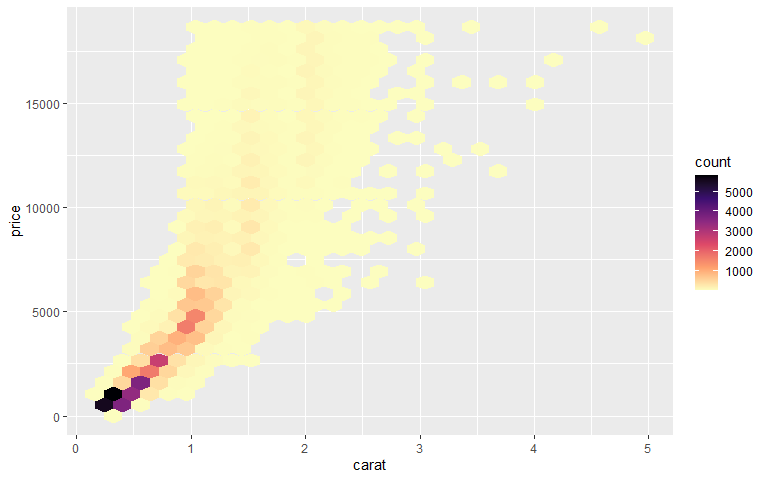``````
ggplot(diamonds, aes(x = carat, y = price)) +
geom_hex() +
scale_fill_viridis_b(option = "magma", direction = -1)``````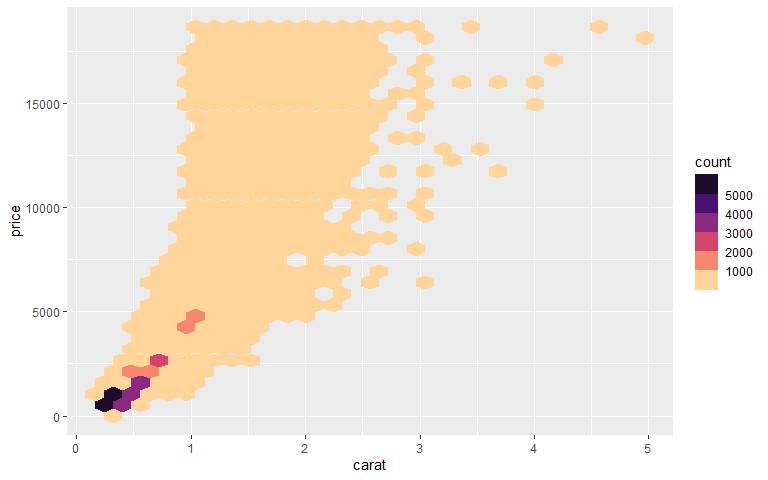``````
ggplot(diamonds, aes(x = carat, y = price)) +
geom_hex(color = "white") +
scale_fill_viridis_b(option = "magma", direction = -1)``````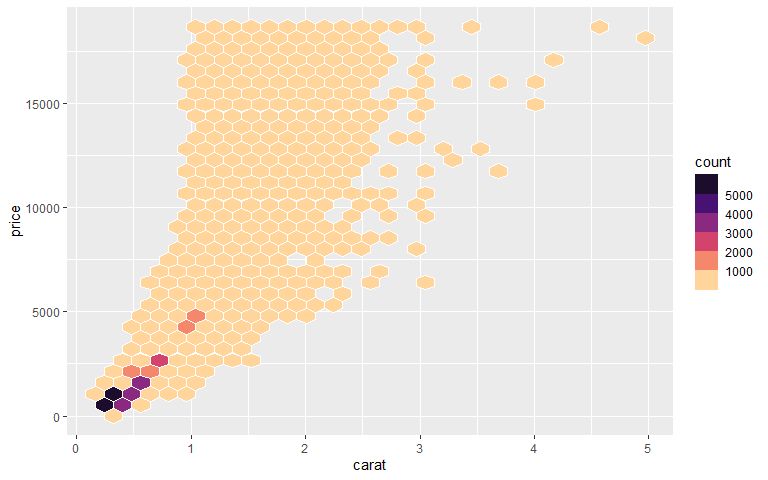``````
ggplot(diamonds, aes(x = carat, y = price)) +
geom_hex(color = "white") +
scale_fill_viridis_b(option = "magma", direction = -1) +
theme_minimal()``````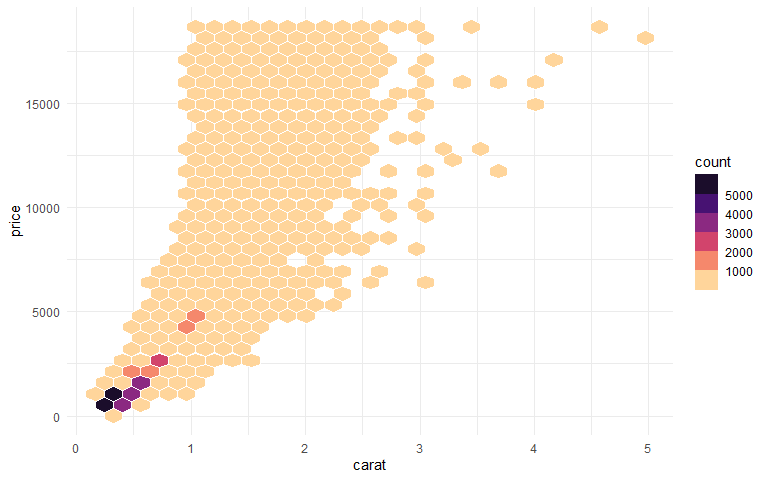``````
ggplot(diamonds, aes(x = carat, y = price)) +
geom_hex(color = "white") +
scale_fill_viridis_b(option = "magma", direction = -1) +
theme_minimal() +
theme(panel.grid.minor = element_blank())``````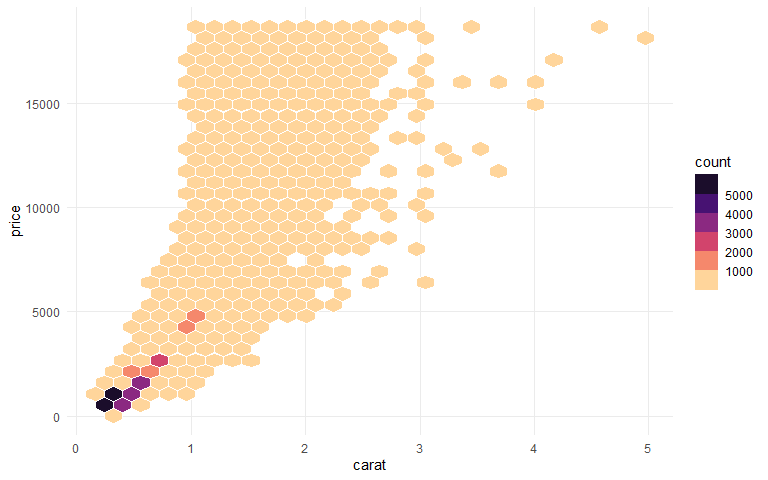``````
ggplot(diamonds, aes(x = carat, y = price)) +
geom_hex(color = "white") +
coord_cartesian(clip = "off") +
scale_y_continuous(labels = scales::dollar_format()) +
scale_fill_viridis_b(option = "magma", direction = -1) +
theme_minimal() +
theme(panel.grid.minor = element_blank()) +
labs(x = "Carat", y = NULL, fill = "Number of diamonds")``````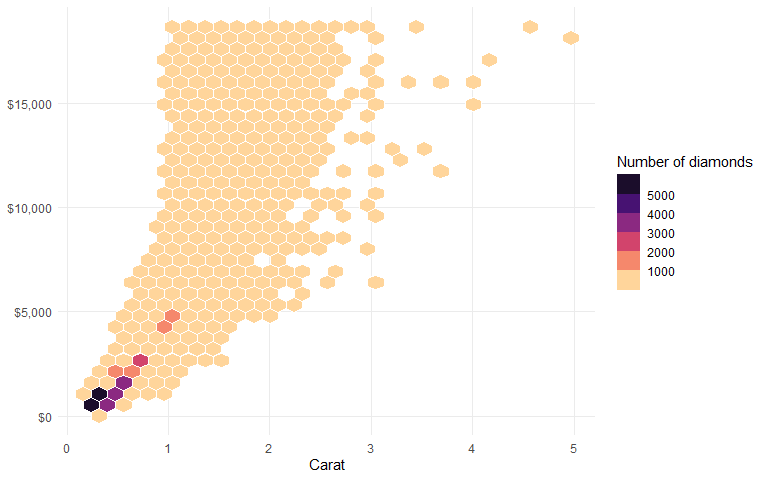``````
ggplot(diamonds, aes(x = carat, y = price)) +
geom_hex(color = "white") +
coord_cartesian(clip = "off") +
scale_y_continuous(labels = scales::dollar_format()) +
scale_fill_viridis_b(option = "magma", direction = -1) +
theme_minimal() +
theme(
panel.grid.minor = element_blank(),
legend.position = "top"
) +
labs(x = "Carat", y = NULL, fill = "Number of diamonds")``````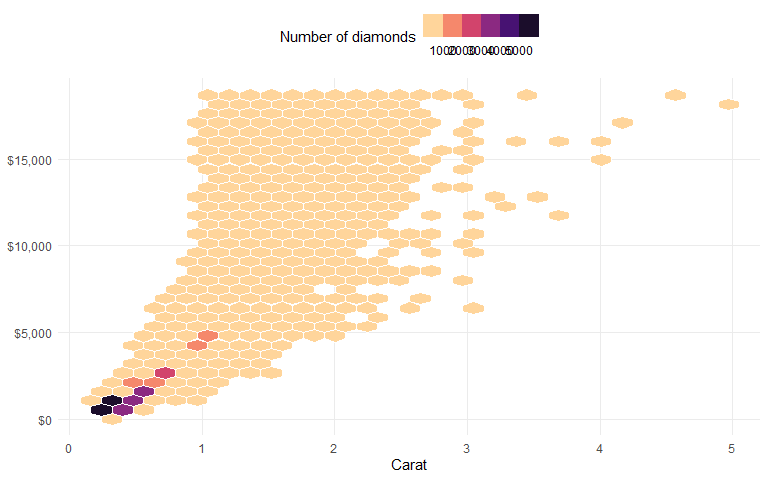``````
g <-
ggplot(diamonds, aes(x = carat, y = price)) +
geom_hex(color = "white") +
coord_cartesian(clip = "off") +
scale_y_continuous(labels = scales::dollar_format()) +
scale_fill_viridis_b(
option = "magma", direction = -1,
guide = guide_colorsteps(
title.position = "top", show.limits = TRUE,
barwidth = unit(16, "lines"), barheight = unit(.8, "lines")
)
) +
theme_minimal() +
theme(
panel.grid.minor = element_blank(),
legend.position = "top"
) +
labs(x = "Carat", y = NULL, fill = "Number of diamonds")

g``````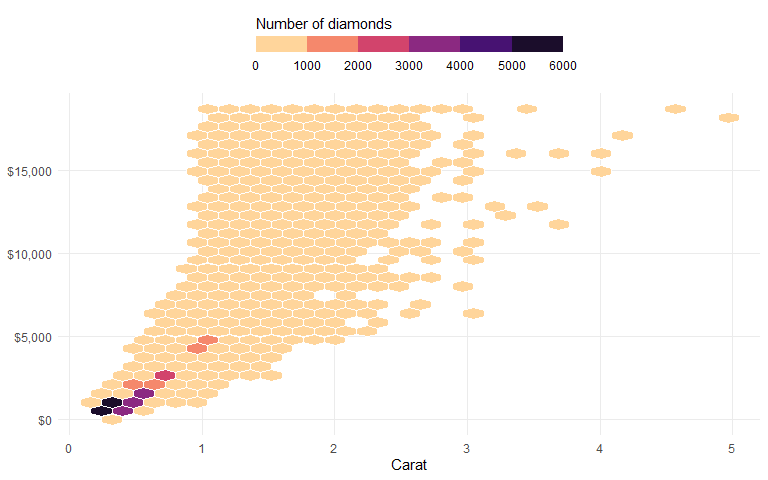## Resize Plots with `gg_resize_film()`

The hex grid looks a bit off, so let’s change the aspect ratio as a final step by calling the `gg_resize_film()` function:

``````gg_resize_film(
height = 5,
width = 5,
units = "in",
dpi = 600
)``````
``g``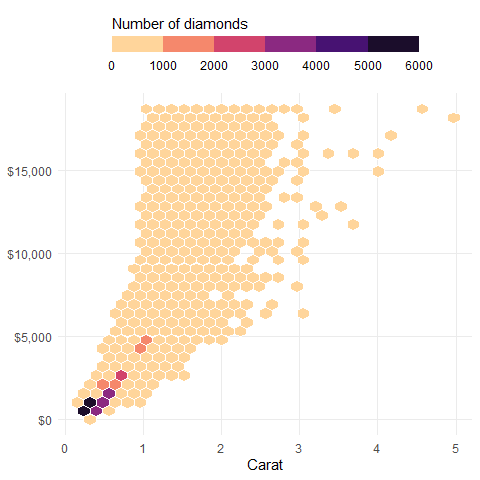## Create a GIF with `gg_playback()`

Once we are happy with the visualization, we can create an animation using all the automatically saved plots:

``````gg_playback(
name = file.path(tempdir(), "recording", "diamonds.gif"),
first_image_duration = 4,
last_image_duration = 12,
frame_duration = .5,
image_resize = 900,
width = 800,
height = 800
)``````

Once rendering is complete, a gif is saved and then opened in your viewer.